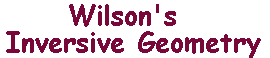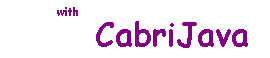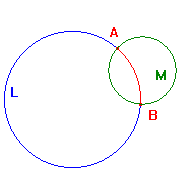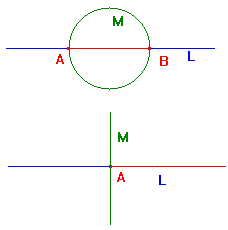The Interior Exterior Theorem

 Suppose that L is an i-line. If L is an extended line, then it divides the rest of E+ into two regions, known as the sides of L. If L is a circle, then it also dvides the rest of E+ into two regions, known as the interior , the region containing the centre, and the exterior the region containing ∞. For the sake of uniformity, we shall also refer to these as sides of the circle. We know that an inversive transformation t maps L to an i-line, but it is not clear that the sides of L map to the sides of t(L). The concept of region is essentially topological, but we wish to give a purely geometrical treatment. The Interior-Exterior Theorem. If L is an i-line and tεI(2), then t maps the sides of L to the sides of t(L). A nice way to show this would be to prove that if two points A and B lie on the same side of an i-line L, then there is an i-line through A and B which does not meet L. Though true, this appears hard to prove withoutrather tedious algebra. In euclidean geometry, the idea of a segment is best described using the idea of betweenness. However, we cannot say that, for three points on an circle, one lies between the other two in any meaningful way. The Interior-Exterior Theorem does allow us to define segments of i-lines in a purely inversive way. Definition If A and B are distinct points on an i-line L, let M be the i-line through A and B orthogonal to L, then the i-segments of L determined by A and B are the intersections of L with the sides of M. We include A and B in both. The existence and uniqueness of such an M is guaranteed by the Uniqueness Theorem. It might appear simpler to take any i-line M through A and B, but then we would have to show that the i-segments so defined do not depend on the choice of M. If L is a circle, this produces the obvious result - the euclidean arcs of L. The top picture shows this case, the i-segements determined by A and B are coloured red and blue. If L is an extended line, then one of the points may be ∞. The middle picture shows a case where A and B are finite. Again, the i-segments are coloured red and blue. the blue i-segment appears to be disconnected, but the two parts "meet" at ∞. The bottom picture shows a case where B is ∞. Once again, the i-segments are coloured red and blue. The point ∞ is on both.After the Interior-Exterior Theorem, it is easy to see that inversive transformations map i-segements to i-segements. We have The i-segment Theorem If A and B lie on an i-line L and tεI(2), then t maps the i-segments of L determined by A and B to the i-segments of t(L) determined by t(A) and t(B).